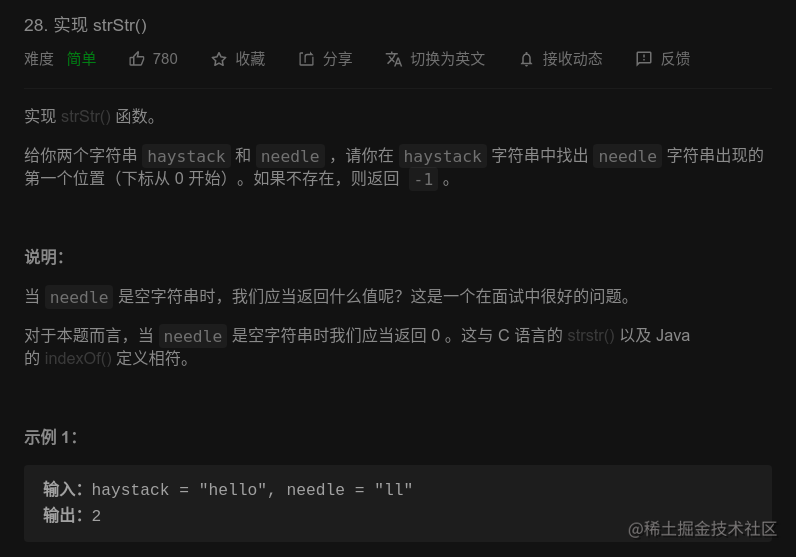# LeetCode 28. 实现 strStr()：看懂 KMP 算法｜刷题打卡

## 一、题目描述：## 二、思路分析：

$s$ 是给定的字符串，而 $p$ 是需要查找的模式串。朴素的字符串匹配会从 $s$ 的某一位 $i$$p$ 的第 $0$ 位开始匹配，如果发现不匹配就退到 $s$$i + 1$ 位和 $p$ 的第 $0$ 位重新开始匹配。

1. 不妨把 $p$ 分成 4 个子串，$prefix \mid mid \mid postfix \mid ch$，其中 $prefix$$p$ 的前缀，$postfix$$p$ 的后缀，它们是相等的，又叫最长公共前后缀，$mid$ 则是它们中间的字符串，$ch$ 表示我们当前要匹配的字符。

2. 如果 $ch$$s$ 中相应位置 $s[i]$ 并不匹配，那么我们无需从 $p$ 开始重新匹配，直接让 $s[i]$$mid$ 所在的位置进行匹配即可，因为 $prefix == postfix$，所以在 $p$ 移动到 $mid$ 后，$prefix$ 势必和 $s[i]$ 前面的字符串匹配。

3. 同理，我们可以用一个 $next$ 数组来记录每个位置不匹配时 $p$ 应该回退的位置，其中 $next[i]$ 表示 $p[0...i - 1]$ 的最长公共前后缀，特别的，$next = -1$，这就是 KMP 算法的核心。注意这里的最长公共前后缀并不是严格意义上的，因为一个字符串也是它本身的前后缀，最长的应该是它本身，这里指的应该是小于它本身的最长公共前后缀。

4. 预处理出 $next$ 数组还需要用到一个技巧，当我们知道 $next[0...i - 1]$ 时如何推出 $next[i]$ 呢? 这个部分我留在了代码的注释里，为了简洁，我在代码注释里使用前缀替代最长公共前缀，后缀亦是如此。

## 三、AC 代码：

class Solution {
private:
vector<int> next;
public:
int strStr(string haystack, string needle) {
buildNext(needle);
return match(haystack, needle);
}

int match(const string &s, const string &p) {
const int n = s.length(), m = p.length();
// 通过 j 的位置来体现匹配是否完成
// 如果 j == m 肯定都匹配完了，所以在下面的循环中无需回退 i
int i = 0, j = 0;
while (i < n && j < m) {
// 当 j = -1 的时候说明当前位置完全不能匹配
// 自然而然地 i 就会 ++ 到下一个位置
if (j < 0 || s[i] == p[j]) {
++i, ++j;
} else {
j = next[j];
}
}
if (j == m) {
return i - j;
}
return -1;
}

void buildNext(const string &p) {
int len = p.length();
if (len == 0) {
return;
}
next = vector<int>(len);
// l 表示前缀末尾的下标，r 则表示后缀末尾的下标
int l = next = -1, r = 0;
while (r < len - 1) {
// 这里的 l，r 是还没 ++ 过的
// 如果 l < 0，表示 p[0...r] 没有公共前后缀，这样会让 next[r + 1] = 0;
// 如果 p[l] == p[r] 就表示公共前后缀的长度可以加 1,而 l + 1 后的结果刚好是前后缀的长度
if (l < 0 || p[l] == p[r]) {
++l, ++r;
next[r] = l;
} else {
// 如果以上条件都不满足，我们就需要缩小已经匹配的前后缀的长度
// 这里让 l = next[l] 的分析和思路分析的第二点类似
l = next[l];
}
}
}
};

## 四、总结：

KMP 算法的思想十分巧妙，但是用代码实现起来不太清晰，虽然很容易看懂，但是实现起来可能会遇到各种问题。不管怎样，最好都自己花时间亲手实现一下，也许可以得到不小的收获。